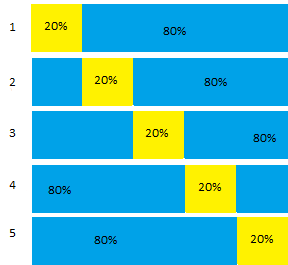# Cross Validation (Statistics)

## What is Cross Validation?In fivefold cross validation, 80% of the data is trained; 20% is tested. the process runs 5 times to cover all of the data.
Cross validation (also called rotation estimation, or out-of-sample testing) is one way to ensure your model is robust. A portion of your data (called a holdout sample) is held back; The bulk of the data is trained and the holdout sample is used to test the model. This is different from the “classical” method of model testing, which uses all of the data to test the model.

Cross validation didn’t become prevalent until huge datasets came into being. Prior to that, analysts preferred to use all the available data to test a model. With larger data sets, it makes sense to hold back a portion of the data to test the model. However, the question becomes which portion of the data do you hold back? Most data isn’t homogeneous across it’s entire length, so if you choose the wrong chunk of data, you could invalidate a perfectly good model. Cross validation solves this problem by using multiple, sequential holdout samples that cover all of the data.

## K-fold Example

In K-fold cross validation (sometimes called v fold, for “v” equal parts), the data is divided into k random subsets. A total of k models are fit, and k validation statistics are obtained.

Let’s say you wanted to run a basic 5-fold algorithm, like the one shown in the above image. The basic procedure is:

1. Set 1/K = 1/5 (20%) of the data aside (chosen randomly).
2. Train the remaining 80% of data.
3. Score the model based on the holdout sample, and record needed model metrics.
4. Restore the holdout sample and then repeat, scoring the next 20% of data.
5. Repeat the procedure until all data has been included in a holdout sample.
6. Find the mean (or similar) of the model metrics.

Leave-one-out cross validation is K-fold with K = N, the number of data points in the set.

## Monte Carlo Cross Validation

Monte Carlo CV works on the same idea as K-Fold, where a percentage of data forms the training set; the rest of data is the test set. The major difference is that with K-fold, all of the data is used exactly once. With Monte Carlo, each holdout sample is chosen independently. For example, let’s say your dataset was made up of 25 points, A-Y.
5-Fold would split the data into:

1. {ABCDE},
2. {FGHIJ},
3. {KLMNO},
4. {PQRST},
5. {UVWXY}.

Monte Carlo would choose 5 points randomly, perhaps {ACWXY}. Those items are place back into the set, and then 5 points are again chosen randomly, with replacement, perhaps {ABKPR}. Item “A” has been chosen twice in this example, which is allowed in Monte Carlo.

Cross validation can be used on data sets ranging from small to large. With smaller data sets, k-fold cross validation makes efficient use of limited data (SAS, 2017). Cross validation is particularly useful for complex models. For example, it could be used on models with iterative processes that respond to local data structures (Bruce & Bruce, 2017).The downside is that the method is computationally expensive.

## References

Bruce, P. & Bruce A. (2017). Practical Statistics for Data Scientists: 50 Essential Concepts. Retrieved May 25, 2019 from: https://books.google.com/books?id=ldPTDgAAQBAJ
Dangeti, P. (2017). Statistics for Machine Learning. Packt Publishing Ltd. Retrieved May 25, 2019 from: https://books.google.com/books?id=C-dDDwAAQBAJ.
SAS (2017). JMP 13 Fitting Linear Models, Second Edition.

CITE THIS AS:
Stephanie Glen. "Cross Validation (Statistics)" From StatisticsHowTo.com: Elementary Statistics for the rest of us! https://www.statisticshowto.com/cross-validation-statistics/
---------------------------------------------------------------------------Need help with a homework or test question? With Chegg Study, you can get step-by-step solutions to your questions from an expert in the field. Your first 30 minutes with a Chegg tutor is free!